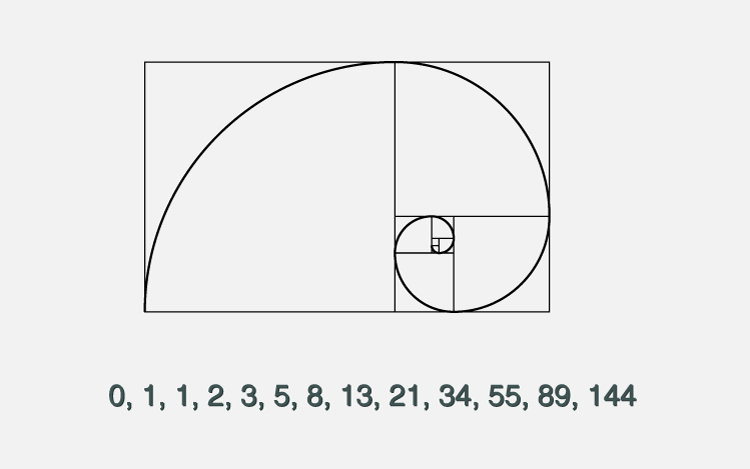# C Program to Display Fibonacci Sequence

In this example, you will learn to display the Fibonacci sequence of first n numbers (entered by the user).To understand this example, you should have the knowledge of the following C programming topics:

The Fibonacci sequence is a sequence where the next term is the sum of the previous two terms. The first two terms of the Fibonacci sequence are 0 followed by 1.

```The Fibonacci sequence: 0, 1, 1, 2, 3, 5, 8, 13, 21
```

## Fibonacci Series up to n terms

``````#include <stdio.h>
int main() {
int i, n, t1 = 0, t2 = 1, nextTerm;
printf("Enter the number of terms: ");
scanf("%d", &n);
printf("Fibonacci Series: ");

for (i = 1; i <= n; ++i) {
printf("%d, ", t1);
nextTerm = t1 + t2;
t1 = t2;
t2 = nextTerm;
}

return 0;
}
``````

Output

```Enter the number of terms: 10
Fibonacci Series: 0, 1, 1, 2, 3, 5, 8, 13, 21, 34,
```

## Fibonacci Sequence Up to a Certain Number

``````#include <stdio.h>
int main() {
int t1 = 0, t2 = 1, nextTerm = 0, n;
printf("Enter a positive number: ");
scanf("%d", &n);

// displays the first two terms which is always 0 and 1
printf("Fibonacci Series: %d, %d, ", t1, t2);
nextTerm = t1 + t2;

while (nextTerm <= n) {
printf("%d, ", nextTerm);
t1 = t2;
t2 = nextTerm;
nextTerm = t1 + t2;
}

return 0;
}
``````

Output

```Enter a positive integer: 100
Fibonacci Series: 0, 1, 1, 2, 3, 5, 8, 13, 21, 34, 55, 89,
```